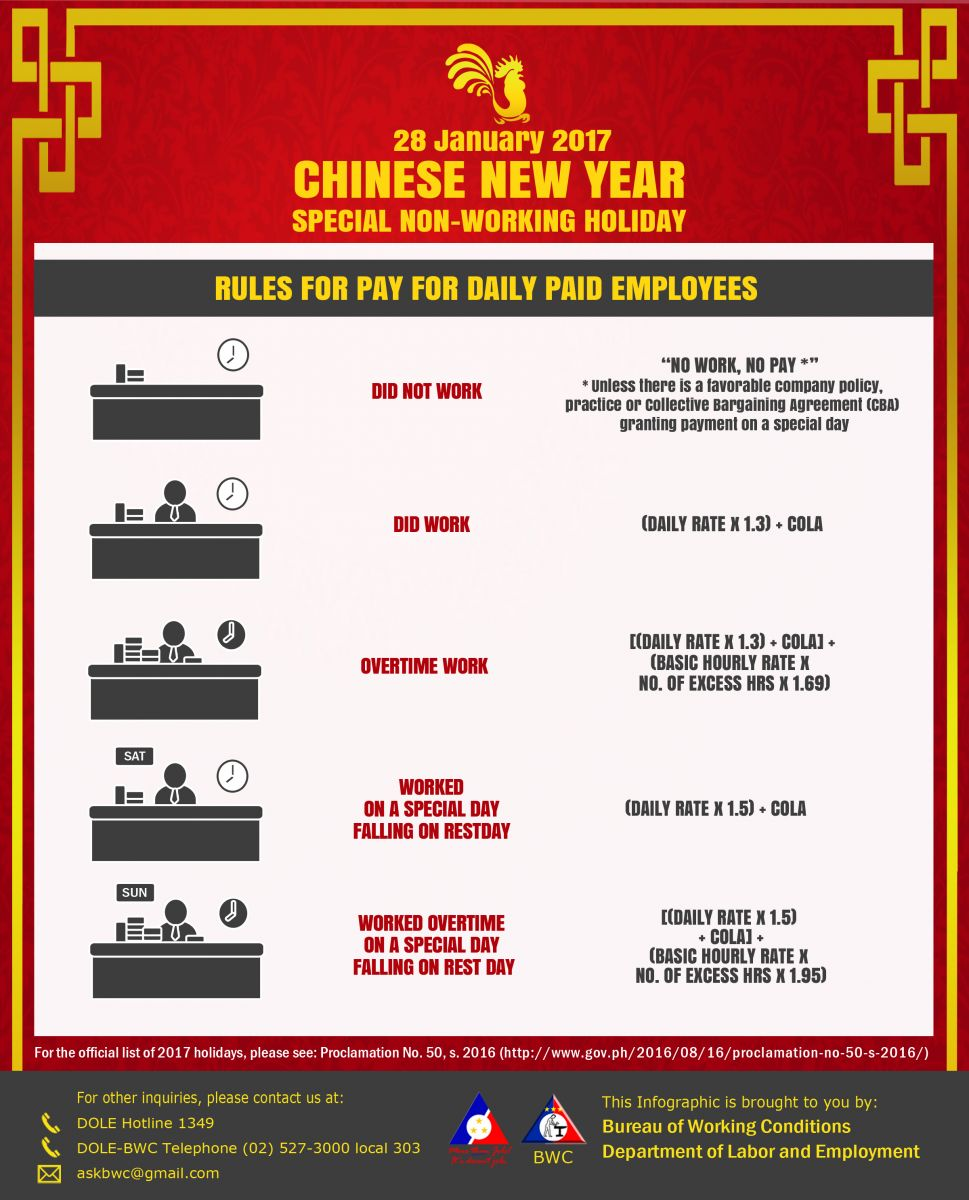# Rules for Pay for the Chinese New Year

You are here:# Rules for Pay for the Chinese New Year

Last January 28, 2017, the country celebrated the Chinese New Year. As it was declared a Special Holiday, special pay rules apply for Daily Paid Employees:

## If you did not work

The No Work, No Pay rule applies – unless there is a favorable company policy, practice or collective bargaining agreement (CBA) granting payment on a special day.

## If you did work

(Daily Rate x 1.3) + COLA

Example: Daily Rate = P500.00, COLA = P15.00
(P500.00 x 1.3) + P15.00 = P665.00

## If you worked Overtime (beyond 8 hours)

[(Daily Rate x 1.3) + COLA] + (Basic Hourly Rate x No. of Excess Hrs x 1.69)

Example: Daily Rate = P500.00, COLA = P15.00, Basic Hourly Rate = P62.50, Excess Hrs = 2
[(P500.00 x 1.3) + P15.00] + (P62.50 x 2 x 1.69) = [P665.00] + (P211.25) = P876.25

## If you worked and it was also your Rest Day

(Daily Rate x 1.5) + COLA

Example: Daily Rate = P500.00, COLA = P15.00
(P500.00 x 1.5) + P15.00 = P765.00

## If you worked overtime (beyond 8 hours) and it was also your Rest Day

[(Daily Rate x 1.5) + COLA] + (Basic Hourly Rate x No. of Excess Hrs x 1.95

Example: Daily Rate = P500.00, COLA = P15.00, Basic Hourly Rate = P62.50, Excess Hrs = 2
[(P500.00 x 1.5) + P15.00] + (P62.50 x 2 x 1.95) = [P765.00] + (P243.75) = P1,008.75